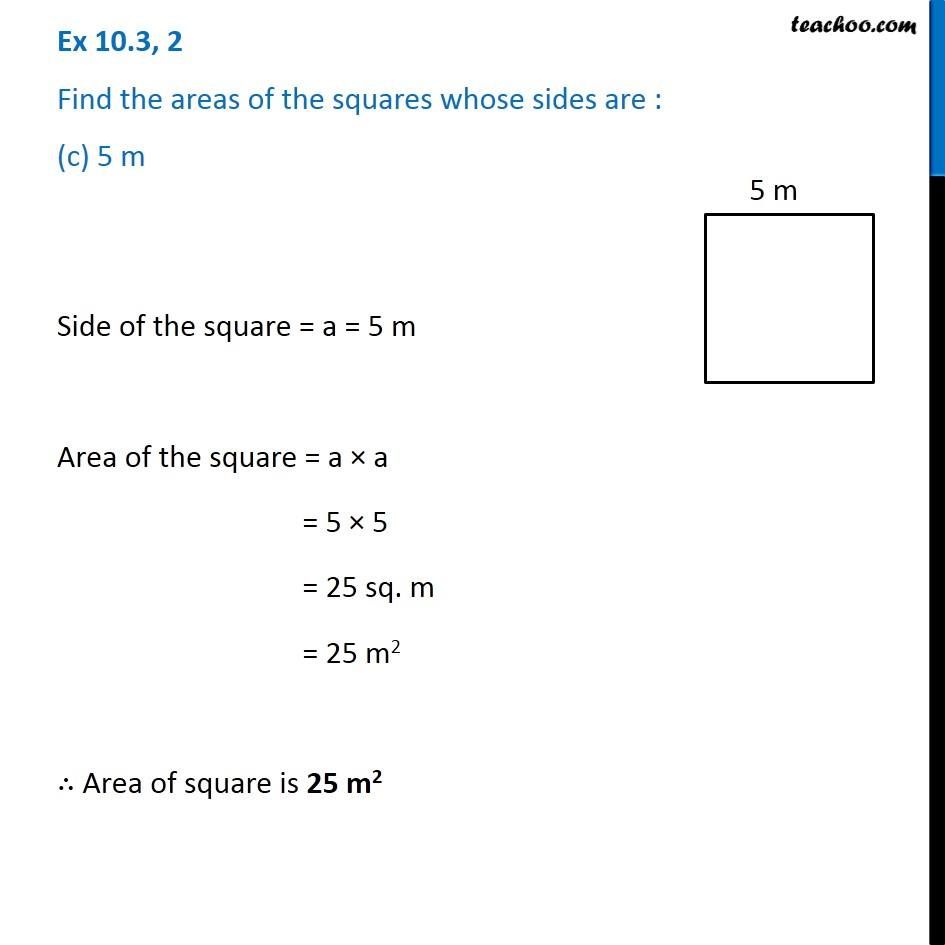1. Chapter 10 Class 6 Mensuration
2. Serial order wise
3. Ex 10.3

Transcript

Ex 10.3, 2 Find the areas of the squares whose sides are : (c) 5 m Side of the square = a = 5 m Area of the square = a × a = 5 × 5 = 25 sq. m = 25 "m2" ∴ Area of square is 25 m2